HomeArticlesProjectsBlogContact
Articles
50 Questions TestColin Mitchell
Make sure to subscribe to our newsletter and be the first to know the news.
```<!-- TODO FIXME: add quizdown here -->
```
```<!-- Begin var ans = new Array; var done = new Array; var score = 0; ans\[1\] = "Light Emitting Diode"; ans\[2\] = "Collector Base Emitter"; ans\[3\] = "A short circuit"; ans\[4\] = "1.7v"; ans\[5\] = "Higher"; ans\[6\] = "4k4"; ans\[7\] = "Higher"; ans\[8\] = "Not enough information -It may be PNP or NPN!"; ans\[9\] = "Load Resistor"; ans\[10\] = "110n"; ans\[11\] = "2k2"; ans\[12\] = "The Emitter"; ans\[13\] = "5k"; ans\[14\] = "PNP Transistor"; ans\[15\] = "0v - The batteries are back-to-back!"; ans\[16\] = "3R3 4R7 22R 5k6"; ans\[17\] = "2k3"; ans\[18\] = "Common Emitter"; ans\[19\] = "Capacitor, Microphone, Potentiometer, Electrolytic"; ans\[20\] = "200n"; ans\[21\] = "Base Bias Resistor"; ans\[22\] = "Timing Circuit/Delay Circuit"; ans\[23\] = "35,200 ohms"; ans\[24\] = "Photo darlington transistor, reed switch, piezo, coil"; ans\[25\] = "Series"; ans\[26\] = "A Tuned Circuit"; ans\[27\] = "Rise"; ans\[28\] = "1n"; ans\[29\] = "0.045Amp"; ans\[30\] = "0.1u"; ans\[31\] = "0.001A"; ans\[32\] = "1.2v"; ans\[33\] = "1mA"; ans\[34\] = "Common Collector/Emitter Follower"; ans\[35\] = "B"; ans\[36\] = "The cathode lead is shorter. It goes to the negative rail"; ans\[37\] = "Between 3 and 35mA, depending on the brightness required"; ans\[38\] = "Inverted"; ans\[39\] = "a"; ans\[40\] = "A"; ans\[41\] = "remains constant"; ans\[42\] = "Cathode Ray Oscilloscope"; ans\[43\] = "microfarad, electrolytic, capacitor, picofarad"; ans\[44\] = "5%, 10%, 1%"; ans\[45\] = "a"; ans\[46\] = "p, n, u"; ans\[47\] = "A Schmitt Trigger"; ans\[48\] = "0.1u or 100n - both are correct"; ans\[49\] = "The voltage across the capacitor"; ans\[50\] = "LOW"; function Try(question, answer) { if (answer != ans\[question\]) { if (!done\[question\]) { done\[question\] = -1; window1=window.open("", "NewWindow1", "toolbar=no,directories=no,menubar=no,scrollbars=no,top=475,left=25,width=320,height=20"); window1.document.writeln("<title>Answers to: 50 QUESTIONS . . . . </title>"); window1.document.writeln("<font face=Arial size=2 color=#FF00cc><center>Wrong!\\n\\nYour score is now: " + score + "<font face=Arial size=2 color=#cc0000><center>The correct answer is: <font face=Arial size=2 color=#000000>" + ans\[question\]); } else { alert("You have already answered that question"); } } else { if (!done\[question\]) { done\[question\] = -1; score++; alert("Correct!\\n\\nYour score is now: " + score); } else { alert("You have already answered that question"); } } } function Assessment () { if (score >= 50) { alert("Perfect Score!"); } if (score >= 45 && score <= 49) { alert("Excellent Work - but not Perfect!") } if (score >= 0 && score <= 44) { alert("You need to do the BASIC ELECTRONICS COURSE. \\nYou will be amazed at how much you will learn.") } } // End -->
```Answer the following … JavaScript is required!
This is a simple test to see how much you know about basic electronics.

```<!-- TODO FIXME WEBMASTER: this test might be duplicated on multiple pages. copy to one locatation & import -->
```

1. What does LED stand for?

• Light Emitting Display
• Low Energy Display
• Light Emitting Diode
• Light Emitting Detector

2. Name the three leads of a common transistor

• Collector Bias Omitter
• Base Collector Case
• Emitter Collector Bias
• Collector Base Emitter

3. Connecting a lead from the negative to the positive of a battery will produce:

• A high resistance circuit
• A short circuit
• A low current path
• An open circuit

4. What is the approximate characteristic voltage that develops across a red LED?

• 1.7v
• 3.4v
• 0.6v
• 5v

5. If two resistors are placed in series, is the final resistance:

• Higher
• Lower
• The same
• Cannot be determined

6. Which is not a “common” value of resistance:

• 2k7
• 1M8
• 330R
• 4k4

7. If a small value of capacitance is connected in parallel with a large value, the combined capacitance will be:

• The same
• Higher
• Lower

8. If the voltage on the base of a transistor increases, does it:

• Turn on
• Turn off
• Not enough information
• Remain the same

9. The resistor identified in brown is called the: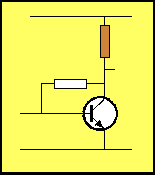• Base Bias Resistor
• Emitter Feedback Resistor
• Bypass Resistor

10. A 100n capacitor in parallel with 10n produces:

• 90n
• 100n
• 110n
• Cannot be determined

11. A resistor with colour bands: red-red-red-gold, has the value:

• 22k 5%
• 2k2 5%
• 220R 5%
• 22R 5%

12. The lead marked with the arrow is: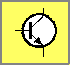• The Collector
• The Base
• The Emitter
• The case

13. A 10k resistor in parallel with 10k produces:

• 10k
• 5k
• 20k
• Cannot be determined

14. The symbol is: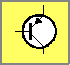• NPN Transistor
• PNP Transistor
• Photo Transistor
• Field Effect Transistor

15. Two 3v batteries are connected as shown. The output voltage is: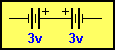• 3v
• 0v
• 6v

16. 4 resistors in ascending order are:

• 22R 270k 2k2 1M
• 4k7 10k 47R 330k
• 3R3 4R7 22R 5k6
• 100R 10k 1M 3k3

17. The closest value for this combination is: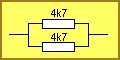• 4k7
• 2k3
• 9k4

18. This stage is called: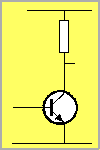• Common Base
• Common Collector
• Common Emitter
• Emitter Follower

19. The four symbols are: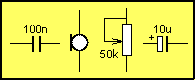• Capacitor, Microphone, Potentiometer, Electrolytic
• Electrolytic, Microphone, Resistor, Capacitor
• Capacitor, Piezo, Resistor, Electrolytic
• Electrolytic, Coil, Resistor, Capacitor

20. The value of the combination is: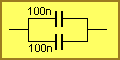• 100n
• 200n
• 50n

21. The resistor marked in red is: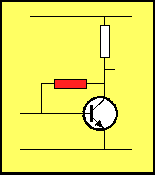• Base Bias Resistor
• Emitter Feedback Resistor
• Bypass Resistor

22. A resistor and capacitor in series is called a: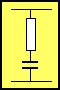• Pulse Circuit
• Timing Circuit/Delay Circuit
• Oscillator Circuit/Frequency Circuit
• Schmitt Circuit

23. A red-red-red-gold resistor in series with an orange-orange-orange-gold resistor produces:

• 5k5
• 35,200 ohms
• 55k
• None of the above

24. Name the 4 components: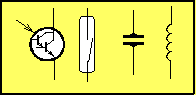• Photo transistor, switch, capacitor, coil
• Transistor, mercury switch, piezo, coil
• Photo transistor, reed switch, piezo, coil
• Photo darlington transistor, reed switch, piezo, coil

25. To obtain a higher value of resistance, resistors are connected in:

• Reverse
• Forward
• Parallel
• Series

26. A capacitor and coil in parallel is called: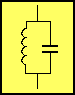• A Tuned Circuit
• A Timing Circuit
• A Delay Circuit
• A Schmitt Circuit

27. When the base is raised, the emitter will: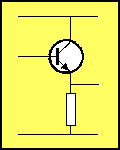• Rise
• Fall
• Remain Fixed
• Oscillate

28. What is 1,000p?

• 0.01n
• 0.0001u
• 0.1n
• 1n

29. The current in a circuit is 45mA. This is:

• 0.045Amp
• 0.00045A
• 0.0045A
• 0.45A

30. A 100n capacitor can be expressed as:

• 0.01u
• 0.001u
• none of the above

31. 1mA is equal to:

• 0.001A
• 0.00001A
• 0.01A
• 0.1A

32. 1,200mV is equal to:

• 12v
• 1.2v
• 0.12v
• 0.0012v

33. If a 10k resistor is placed across a 10v supply, the current will be:

• 10mA
• 1mA
• 0.01mA
• 0.1mA

34. This arrangement is called:• Common Emitter
• Common Collector/Emitter Follower
• Common Base

35. Identify the correctly connected LED: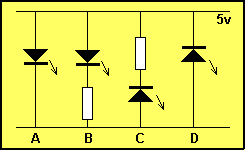• A
• B
• C
• D

36. Identify the correct statement: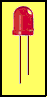• The cathode lead is longer. It goes to the negative rail
• The cathode lead is shorter. It goes to the negative rail
• The cathode lead is shorter. It goes to the positive rail
• The cathode lead is longer. It goes to the positive rail

37. The current requirement of a LED is:

• 1.7mA
• 25mA
• Between 3 and 35mA
• 65mA

38. The signal at the collector will be …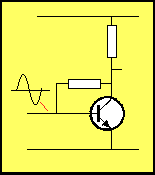• Inverted …
• In-phase …
• . . .with the base.

39. The purpose of the capacitor: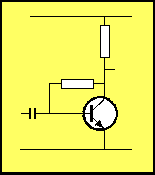• To pass AC on the input to the base
• To allow the transistor to self-bias
• Block DC from the input line
• To allow the stage to operate

40. The direction of conduction for a diode is: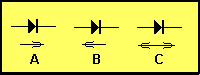• A
• B
• C

41. A DC voltage …

• rises and falls
• is a sinewave
• remains constant
• is an audio waveform

42. A CRO is a

• Cathode Ray Oscillator
• Cathode Ray Oscilloscope
• Capacitor-Resistor Oscillator
• Capacitor-Resistor Output

43. These jargon terms mean: (Jargon = language peculiar to a ‘mickey’ ‘electro’ ‘cap’ ‘puff’; trade)

44. The tolerance bands: gold; silver; brown, represent:

• 10%, 5%, 1%
• 5%, 10%, 2%
• 5%, 10%, 1%
• 10%, 5%, 2%

45. 223 on a capacitor represents:

• All of the above

46. Arrange these in ascending order: n, p, u

• p, u, n,
• n, u, p
• p, n, u

47. Name this symbol: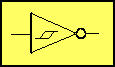• A buffer
• A NOR gate
• A NAND gate
• A Schmitt Trigger

48. The number “104” on a capacitor indicates:

• 0.1u
• 100n
• 1n
• 10n

49. What is the multimeter detecting: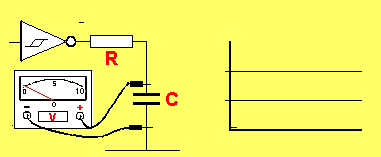• The output voltage of the Schmitt Trigger
• The delay across the capacitor
• The voltage across the capacitor
• The current through the capacitor

50. For the XOR gate, what is the output when both inputs are HIGH: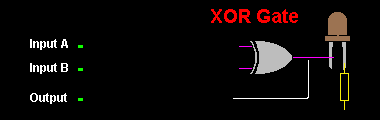• HIGH
• LOW
• Can be HIGH or LOW
• Cannot be determined

These are the types of questions you should be able to answer INSTANTLY, if you want to design and build microcontroller projects. If you get less than 45, now’s the time to do the Basic Electronics course. Try the test again after the course, I’m sure you will “fly” through the answers and be amazed at how much you have learnt.

electronics
writing
PIC-Chips